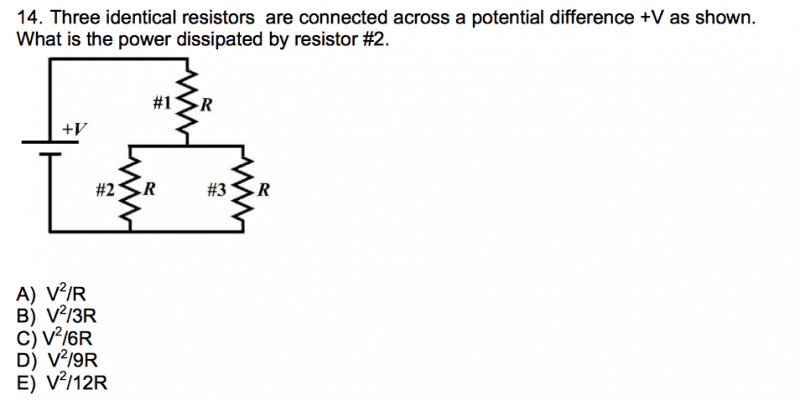# Power dissipated by a resistor in a circuit

## Homework Statement## Homework Equations

P = V^2 / R
1/Rt = sum(1/Ri) (parallel)

## The Attempt at a Solution

[/B]
Since all the answer choices express the solution in terms of V^2 / R, I tried to find the voltage drop across resistor #2 to find the power. I collapsed the two parallel resistors 2 and 3 into one and found their effective resistance to be R/2 (parallel formula). Using Kirchhoff's voltage law,

V = IR + I(R/2) = 3IR / 2 where I is the total current in the circuit

Since V = 3IR/2, I can rewrite the voltage drop across the collapsed resistors as (⅓)V. Therefore the voltage drops across resistors #2 and #3 are both (⅓)V since they are in parallel. Plugging into the power formula,

P = (1/3V)^2 / R = V^2 / 9R, answer choice D. But the answer key says the solution is B. Why?

TSny
Homework Helper
Gold Member
Your reasoning looks correct to me. I agree that D is the correct answer. Apparently the answer key is wrong.

CWatters